# 7.2: Government expenditure and taxes

$$\newcommand{\vecs}{\overset { \rightharpoonup} {\mathbf{#1}} }$$ $$\newcommand{\vecd}{\overset{-\!-\!\rightharpoonup}{\vphantom{a}\smash {#1}}}$$$$\newcommand{\id}{\mathrm{id}}$$ $$\newcommand{\Span}{\mathrm{span}}$$ $$\newcommand{\kernel}{\mathrm{null}\,}$$ $$\newcommand{\range}{\mathrm{range}\,}$$ $$\newcommand{\RealPart}{\mathrm{Re}}$$ $$\newcommand{\ImaginaryPart}{\mathrm{Im}}$$ $$\newcommand{\Argument}{\mathrm{Arg}}$$ $$\newcommand{\norm}{\| #1 \|}$$ $$\newcommand{\inner}{\langle #1, #2 \rangle}$$ $$\newcommand{\Span}{\mathrm{span}}$$ $$\newcommand{\id}{\mathrm{id}}$$ $$\newcommand{\Span}{\mathrm{span}}$$ $$\newcommand{\kernel}{\mathrm{null}\,}$$ $$\newcommand{\range}{\mathrm{range}\,}$$ $$\newcommand{\RealPart}{\mathrm{Re}}$$ $$\newcommand{\ImaginaryPart}{\mathrm{Im}}$$ $$\newcommand{\Argument}{\mathrm{Arg}}$$ $$\newcommand{\norm}{\| #1 \|}$$ $$\newcommand{\inner}{\langle #1, #2 \rangle}$$ $$\newcommand{\Span}{\mathrm{span}}$$

A basic government budget has two components:

1. A plan for government expenditures on goods and services, G.
2. A net tax rate on income, t, set to generate revenue to finance expenditure.

When added to the definition of autonomous expenditure, A is expanded to include government expenditure G.

Government expenditure (G): government spending on currently produced goods and services.

We illustrate autonomous government expenditure in the same way we did with other autonomous expenditures, using a simple equation. For a specific level of government expenditure:(7.1)

In Figure 7.1 with income on the horizontal axis and government expenditure and net tax revenue on the vertical axis, a horizontal line intersecting the vertical axis at G0 illustrates a particular level of government expenditure. Any change in government expenditure would shift this line up or down in a parallel way.

Figure 7.1 Government expenditure and net tax revenue functionThe government raises revenue by levying direct taxes on incomes and pays out transfer payments such as old age security, employment insurance benefits, social assistance and interest on the public debt. The difference between taxes collected and transfers paid is net taxes (NT), the net revenue collected by government from households.

Net taxes: taxes on incomes minus transfer payments.Figure 7.1 illustrates another important point. It shows that for a given level of planned government expenditure and a given tax rate, government expenditure will be greater than tax revenue at lower income levels and lower than tax revenue at higher income levels. There is a strong direct linkage between government finances and national income.

There is another but slightly more complex link between government taxes and non-government expenditures. The net tax rate on income reduces induced consumption. Net taxes reduce disposable income—the amount available to households for spending or saving—relative to national income. If YD is disposable income, Y national income and output, and NT net taxes: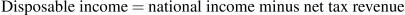(7.2)

Disposable income (YD): national income minus net taxes.

Suppose taxes net of transfers are about 15 percent of national income. The net tax rate t=0.15. If national income Y increases by $1, net tax revenue will increase by$0.15, but household disposable income will increase by only $0.85. For simplicity, suppose the marginal propensity to consume (mpc=c) out of disposable income is 0.8. The consumption function is: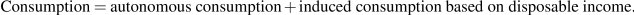Then if: C=20+0.8YD And the net tax rate is t=0.15, disposable income is (1–0.15)=0.85 times national income. Thus, consumption expenditure based on national income is:  CC =20+0.68Y A change in national income of$1 changes consumption expenditure by only 0.8 times (1–t) of a dollar. If the net tax rate is 0.15, consumption expenditure changes by only. Each extra dollar of national income increases disposable income by $0.85, out of which households plan to spend 68 cents and save 17 cents. Table 7.3 gives a numerical example. Table 7.3 A numerical example  a) C=20+0.8YD b) C=20+0.8YD NT=0 NT=0.15Y YD=Y YD=(1–0.15)Y C=20+0.8(1–0.15)Y a) Y NT YD C1 b) Y NT YD C2 100 0 100 100 100 15 85 88 300 0 300 260 300 45 255 224 500 0 500 420 500 75 425 360 In the absence of taxation, in part (a) of the table, national income Y and disposable income YD are the same. The consumption function C1 shows how much households wish to consume at each level of national income, based on the numerical example. With a proportional net tax rate of 0.15, households still consume$0.80 of each dollar of disposable income. Now part (b) shows YD is now only 0.85 of Y when the net tax rate is 0.15. Households consume onlyof each extra dollar of national income.

## The effects of both government expenditure and taxation

Aggregate expenditure includes both autonomous and induced expenditure. The net tax rate diverts some of national income to the government budget and lowers the disposable income on which induced expenditure is based. Net Taxes and imports both lower the marginal propensity to spend on domestic output. With the marginal propensity to consume out of disposable income (mpc), the marginal propensity to import (mpm) and the net tax rate (t), the slope of the AE function, the marginal propensity to spend on domestic output is: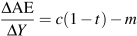(7.3)
Figure 7.2 The effect of G and NT on AENew government expenditure and taxes shift the AE function up by G0 and lower its slope from (cm) to c(1–t)–m. A change in G shifts AE vertically. A change in t changes the slope of AE.

Figure 7.2 shows the combined effect of government expenditure and net taxes on the aggregate expenditure function.

As in the basic model, equilibrium real GDP equals autonomous aggregate expenditure multiplied by the multiplier. The government sector adds a new autonomous expenditure component G0 that shifts the AE function, and a new induced expenditure factor, the net tax rate (t), which lowers the slope of the AE function. Figure 7.3 illustrates the effects on equilibrium GDP and the multiplier.

Figure 7.3 Government expenditure, taxes, and equilibrium real GDP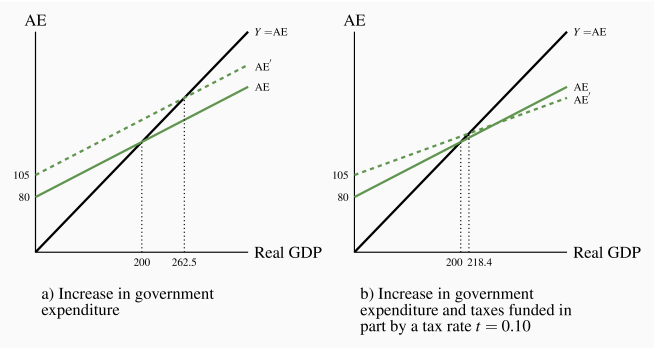Part a) of Figure 7.3 shows the effect of adding government expenditure to aggregate expenditure in the basic model. Government expenditure G=25 is autonomous and shifts the AE curve up to a new intercept at 105. With mpc=0.8 and mpm=0.2 the slope of AE is 0.6 and the multiplier is 2.5. Equilibrium Y increases byto the new equilibrium 262.5. An increase in autonomous government expenditure increased equilibrium GDP. A cut in government expenditure would reduce it. In this example, since the government has not introduced a tax system the government expenditure is financed by borrowing money through the sale of bonds.

## The multiplier revisited

The multiplier relates changes in equilibrium national income to the changes in autonomous expenditures that cause them. The formula in Chapter 6 still applies.Without government and taxes, disposable income and national income are the same. With induced expenditure based on the marginal propensity to consume (mpc=c) and the marginal propensity to import (mpm=m) the multiplier is:With government net taxes proportional to income, NT=tY, disposable income is less than national income namely YtY. This reduces the marginal propensity to consume out of national to c(1–t), reducing the slope of the AE function.As a result, the multiplier, which is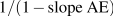is smaller: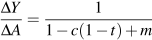(7.4)

The numerical values in Figure 7.3 provide one example of this change in the multiplier.

Now that we have seen that government expenditure and net tax taxes have effects on aggregate expenditure and equilibrium income, it is time to examine the effects of government budgets on AE, AD, and real GDP. The government implements fiscal policy through its budget.

Application Box 7.1 The multiplier in Canada

The multiplier plays a key role in the AE and AD/AS model of the economy. But what is the size of the multiplier in Canada? A simple statistical estimate, using Statistics Canada annual data for real GDP and consumption expenditures, gives a Canadian marginal propensity to consume out of national income c(1–t)=0.54, and marginal propensity to import m=0.34. Using these estimates, we get a multiplier for Canada:Recent data gives an estimate of the Canadian marginal propensity to consume out of disposable income of mpc=0.88. If there were no taxes or imports, an mpc=0.88 would mean a multiplier of about 8.33. The difference between the multipliers 1.25 and 8.33 shows clearly the automatic stabilization coming from the net tax rate and marginal propensity to import.

This page titled 7.2: Government expenditure and taxes is shared under a CC BY-NC-SA license and was authored, remixed, and/or curated by Douglas Curtis and Ian Irvine (Lyryx) .